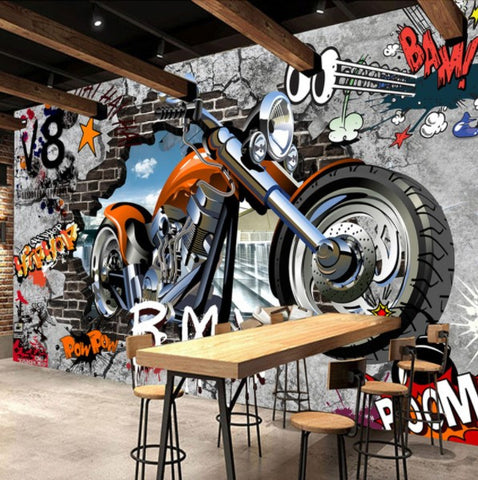## Graffiti Street Art Large Motorcycle Wallpaper for Home or Business

• \$39.95

Product Details

• Features: Waterproof, Smoke-Proof, Moisture-Proof, Fireproof, Mold Proof, Soundproof, Sound-Absorbing, Heat Insulation, Anti-static, Mildew Resistant, Extra Thick, Environment friendly.
• Pattern: Graffiti Street Art Motorcycle
• Surface Treatment: Embossed
• Type: Non-woven, Wood fiber

IMPORTANT PLEASE READ BEFORE BUYING:  DO NOT BUY ONLY 1 PIECE IF YOU WANT TO COVER A FULL WALL.  ONE PIECE is very small, only about 4'7"x 2'4".  The example pictures show a fully covered wall so you know what it will look like if you buy enough pieces to cover it.  A full wall needs many more pieces than just 1.  Pictures are EXAMPLES only.   You need to measure your wall and select how many pieces you need to purchase. There are fixed sizes listed below for you to choose from OR if you need help with calculations just let us know.  We would be happy to help you.

How to calculate how many pieces you need:

Measure your wall.  For example, if your wall is 360cm width x 240cm height, multiply

(360) x (240) = 8.9cm² = 9cm².  So you would need to order 9 pieces.

Here are some fixed sizes:

Quantity 1  :  1 square meter = 140cm(W) x 70cm(H) (4'7" x 2'4") ft = \$49.95

Quantity 2  :  2 square meter = 200cm(W) x 100cm(H) (6'7" x 3'3") ft = \$99.90

Quantity 3  :  3 square meter = 220cm(W) x 140cm(H) (7'3" x 4'7") ft = \$149.85

Quantity 4  :  4 square meter = 250cm(W) x 160cm(H) (8'2" x 5'3") ft = \$199.80

Quantity 5  :  5 square meter = 280cm(W) x 180cm(H) (9'2" x 5'11") ft = \$249.75

Quantity 6  :  6 square meter = 300cm(W) x 200cm(H) (9'10" x 6'7") ft = \$299.70

Quantity 7  :  7 square meter = 330cm(W) x 210cm(H) (10'10" x 6'11") ft = \$349.65

Quantity 8  :  8 square meter = 360cm(W) x 230cm(H) (11'10" x 7'6") ft = \$399.60

Quantity 9  :  9 square meter = 380cm(W) x 240cm(H) (12'5" x 7'10") ft = \$449.55

Quantity 10 :  10 square meter = 400cm(W) x 250cm(H) (13'1" x 8'2") ft = \$499.50

Quantity 11 : 11 square meter = 420cm(W) x 260cm(H) (13'9'' x 8'6'') ft = \$549.45

Quantity 12 : 12 square meter = 440cm(W) x 270cm(H) (14'5" x 8'10") ft = \$599.40

Quantity 13 : 13 square meter = 460cm(W) x 280cm(H) (15'1'' x 9'2'') ft = \$649.35

Quantity 14 : 14 square meter = 480cm(W) x 290cm(H) (15'9'' x 9'6'') ft = \$699.30

Quantity 15 : 15 square meter= 500cm(W) x 300cm(H) (16'5'' x 9'10'') ft = \$749.25

Quantity 16 : 16 square meter=500cm(W) x 320cm(H) (16'5" x 10'6") ft = \$799.20

We Also Recommend